RS Aggarwal Class 10 Solutions Chapter 18 - Areas Of Circle Sector And Segment Ex 18B (18.2)

RS Aggarwal Class 10 Chapter 18 - Areas Of Circle Sector And Segment Ex 18B (18.2) Solutions Free PDF

Question 1: In the given figure, APB and AQO are semi-circles and AO = OB. If the perimeter of the figure is 40 cm, find the area of the shaded region.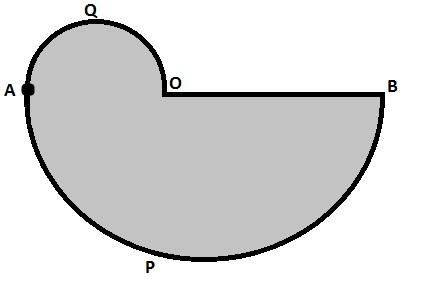Solution:

Perimeter of shaded region = Length of the arc AQO + Length of the arc APB + Length of OB

$\Rightarrow$ 40 = $\frac{1}{2}\times 2\Pi \left ( \frac{AO}{2} \right )+\frac{1}{2}\times 2\Pi (OB)+OB$

$\Rightarrow$ 40 = $\frac{11}{7}AO+\frac{22}{7}OB+OB$

$\Rightarrow$ 40 = $\frac{11}{7}OB+\frac{22}{7}OB+OB$ [Since, AO = OB]

$\Rightarrow$ 40 = $\frac{40}{7}OB$

$\Rightarrow$ OB = 7 cm

Area of the shaded portion = Area of semicircle AQO + Area of semicircle APB

= $\frac{1}{2}\Pi \left ( \frac{7}{2} \right )^{2}+\frac{1}{2}\Pi (7)^{2}$

= $\frac{1}{2}\times \frac{22}{7}\times \left ( \frac{7}{2} \right )^{2}+\frac{1}{2}\times \frac{22}{7}\times (7)^{2}$ = 96.25 cm2

Hence, the area of the shaded portion is 96.25 cm2.

Question 2: Find the area of a quadrant of a circle whose circumference is 44 cm.

Solution:

Let the radius of the circle be r.

Now,

Circumference = 44

$\Rightarrow$ $2\Pi r$ = 44

$\Rightarrow$ r = 7 cm

Now,

Area of quadrant = $\frac{1}{4}\Pi r^{2}=\frac{1}{4}\times \frac{22}{7}\times (7)^{2}=38.5cm^{2}$

Hence, the area of the quadrant of the circle is 38.5 cm2.

Question 3: In the given figure, find the area of the shaded region, where ABCD is a square of side 14 cm and all circles are of the same diameter.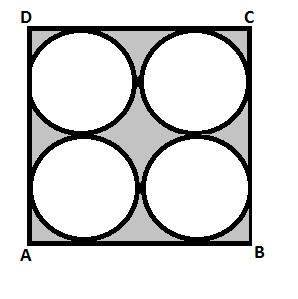Solution:

Area of the square = Side2 = 142 = 196 sq. cm

Area of the circle = 4 x $\Pi$ x 3.5 x 3.5 = 154 sq. cm

Area of the shaded region = Area of the square – Area of four circles = 196 – 154 = 42 cm2

Question 4: Find the area of the shaded region in the given figure, if ABCD is a rectangle with sides 8 cm and 6 cm and O is the centre of the circle.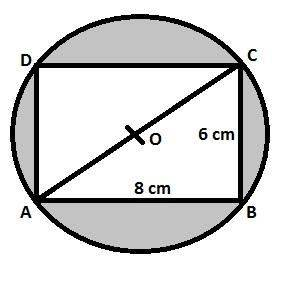Solution:

In right triangle ABC

AC2 = AB2 + BC2 = 82 + 62 = 64 + 36 = 100

Therefore, AC2 = 100

$\Rightarrow$ AC = 10 cm

Now, Radius of circle (OA) = $\frac{1}{2}$ AC = 5 cm

Area of the shaded region = Area of circle – Area of rectangle OABC

= $\Pi (OA)^{2}-AB\times BC$ = $\frac{22}{7}\times (5)^{2}-8\times 6$ = 78.57 – 48 = 30.57 cm2

Hence, the area of the shaded region is 30.57 cm2.

Question 5: A wire is bent to form a square enclosing an area of 484 m2. Using the same wire, a circle is formed. Find the area of the circle.

Solution:

Area of the circle = 484 cm2

Area of the square = Side2

$\Rightarrow$ 484 = Side2

$\Rightarrow$ 222 = Side2

$\Rightarrow$ Side = 22 cm

Perimeter of the square = 4 x Side

Perimeter of the square = 4 x 22 = 88 cm

Length of the wire = 88 cm

Circumference of the circle = Length of the wire = 88 cm

Now, let the radius of the circle be r cm.

Thus, we have:

$\Rightarrow$ 2 x $\frac{22}{7}$ x r = 88

$\frac{22}{7}$ r = 14

Area of the circle = $\Pi r^{2}$ = $\frac{22}{7}$ x 14 x 14 = 616 cm2

Thus, the area enclosed by the circle is 616 cm2.

Question 6: A square ABCD is inscribed in a circle of radius r. Find the area of the square.

Solution:

Let the diameter of the square be d and having circumscribed circle of radius r.

We know that if a circle circumscribes a square, then the diameter of the circle is equal to the diagonal of the square.

Therefore, d = 2r

Now,

Area of square = $\frac{1}{2}d^{2}$ = $\frac{1}{2}(2r)^{2}$ = 2r2 sq. units

Hence, the area of the square ABCD is 2r2 sq. units.

Question 7: The cost of fencing a circular field at the rate of Rs. 25 per metre is Rs. 5500. The field is to be ploughed at the rate of 50 paise per m2. Find the cost of ploughing the field. [Take $\Pi =\frac{22}{7}$

Solution:

Circumference = $\frac{Total\, cost\, of\, fencing}{Rate\, of\, fencing}=\frac{5500}{25}=220$

Let the radius of the circle be r.

Now,

Circumference = 220

$\Rightarrow$ $2\Pi r$ = 220

$\Rightarrow$ r = 35 cm

Now,

Area of field = $\Pi r^{2}$ = $\frac{22}{7}\times (35)^{2}=3850 cm^{2}$

Cost of plugging = Rate x Area of field = 0.5 x 3850 = Rs 1925

Hence, the cost of plugging the field is Rs 1925.

Question 8: A park is in the form of a rectangle 120 m by 90 m. At the centre of the park, there is a circular lawn as shown in the figure. The area of the park excluding the lawn is 2950 m2. Find the radius of the circular lawn. (Given, $\Pi =3.14$)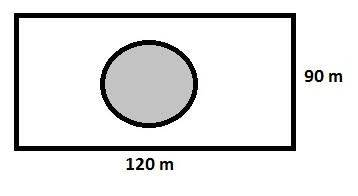Solution:

Area of the rectangle = l x b = 120 x 90 = 10800 sq. m

Area of the park excluding the lawn = 2950 m2

Area of the circular lawn = Area of the park – Area of the park excluding the lawn = 10800 – 2950 = 7850 m2

Area of the circular lawn = $\Pi r^{2}$

$\Rightarrow$ 7850 = $\frac{22}{7}\times r^{2}$

$\Rightarrow$ $\frac{7850\times 7}{22}=r^{2}$

$\Rightarrow$ r2 = 2497.72

$\Rightarrow$ r = 49.97

Or,

r $\approx$ 50 m

Thus, the radius of the circular lawn is 50 m.

Question 9: In the given figure, PQSR represents a flower bed. If OP = 21 m and OR = 14 m, find the area of the flower bed.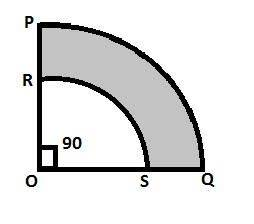Solution:

Area of the flower bed is the difference between the areas of sectors OPQ and ORS.

Area of the flower bed = $\frac{\Theta }{360}\times \Pi (PO^{2}-OR^{2})$

= $\frac{90}{360}\times \frac{22}{7}(21^{2}-14^{2})$ = $\frac{1}{4}\times \frac{22}{7}\times 35\times 7$ = 192.5 m2

Question 10: In the given figure, O is the centre of the bigger circle, and AC is its diameter. Another circle with AB as diameter is drawn. If AC = 54 cm and BC = 10 cm, find the area of the shaded region.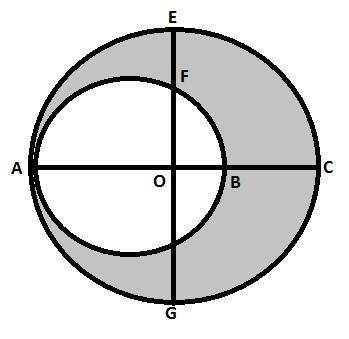Solution:

We have:

OA = OC = 27 cm

AB = AC – BC = 54 – 10 = 44

AB is the diameter of the smaller circle.

Thus, we have:

Radius of the smaller circle = $\frac{AB}{2}$ = $\frac{44}{2}$ = 22 cm

Area of the smaller circle = $\Pi r^{2}$ = $\frac{22}{7}\times 22\times 22$ = 1521.14 cm2

Radius of the larger circle = $\frac{AC}{2}$ = $\frac{54}{2}$ = 27 cm

Area of the larger circle = $\Pi r^{2}$

= $\frac{22}{7}\times 27\times 27$ = 2291.14 cm2

Therefore, Area of the shaded region = Area of the larger circle – Area of the smaller circle = 2291.14 – 1521.14 = 770 cm2

Question 11: From a thin metallic piece in the shape of a trapezium ABCD in which AB || CD and $\angle$BCD = 90o, a quarter circle BFEC is removed. Given, AB = BC = 3.5 cm and DE = 2 cm, calculate the area of remaining (shaded) part of metal sheet.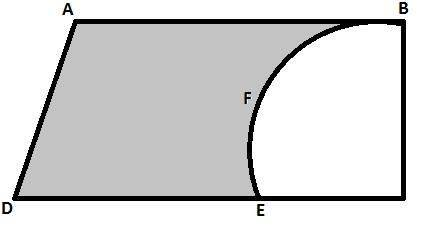Solution:

Since, BFEC is a quarter of a circle.

Hence, BC = EC = 3.5 cm

Now, DC = DE + EC = 2 + 3.5 = 5.5 cm

Area of shaded region = Area of the trapezium ABCD – Area of the quadrant BFEC

= $\frac{1}{2}\times (AB+DC)\times BC-\frac{1}{4}\times \Pi (EC)^{2}$

= $\frac{1}{2}\times (3.5+3.5)\times 3.5-\frac{1}{4}\times \frac{22}{7}\times (3.5)^{2}$ = 6.125 cm2

Hence, the area of the shaded region is 6.125 cm2.

Question 12: Find the area of the major segment APB of a circle of radius 35 cm and $\angle$AOB = 90o, as shown in the given figure.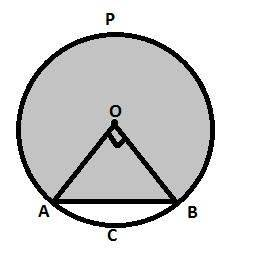Solution:

Area of minor segment = Area of sector AOBC – Area of right triangle AOB

= $\frac{90}{360}\times \Pi (OA)^{2}-\frac{1}{2}\times OA\times OB$

= $\frac{1}{4}\times \frac{22}{7}\times (35)^{2}-\frac{1}{2}\times 35\times 35$ = 962.5 – 612.5 = 350 cm2

Area of major segment APB = Area of circle – Area of minor segment

= $\Pi (OA)^{2}-350$ = $\frac{22}{7}\times (35)^{2}-350$ = 3850 – 350 = 3500 cm2

Hence, the area of major segment is 3500 cm2.

Exercise – 18B

Let $\Pi = \frac{22} {7}$ unless stated otherwise.

Q.1: The circumference of a circle is 39.6 cm. Find its area.

Sol:

Circumference = 39.6 cm

We know,

Circumference of a circle = $2 \Pi r$

$\Rightarrow 39.6 = 2 \times \frac{22}{7}\times r$

$\Rightarrow \frac{39.6 \times 7}{2 \times 22} = r$

$\Rightarrow r= 6.3$ cm

Also,

Area of the circle = $\Pi r^{2}$

$= \frac{22}{7} \times 6.3 \times 6.3$ = $124.74 cm^{2}$

Q.2: The area of a circle is $98.56 cm^{2}$. Find its circumference.

Sol:

Now,

Area = 98.56

$\Rightarrow \pi r^{2} = 98.56$

$\Rightarrow r = 5.6$

Now,

Circumference = $= 2 \pi r = 2 \times \frac{22}{7} \times 5.6 = 35.2$ cm

Hence the circumference of the circle is 35.2 cm.

Q.3: The circumference of a circle exceeds its diameter by 45 cm. Find the circumference of the circle.

Sol:

Let the radius of the circle be r.

Now,

Circumference = Diameter +45

$\Rightarrow 2 \pi r = 2 r + 45$

$\Rightarrow 2 \pi r – 2 r = 45$

$\Rightarrow 2r (\frac{22}{7} – 1) = 45$

$\Rightarrow 2r (\frac{15} {7} ) = 45$

$\Rightarrow r = \frac{45\times 7}{30} = 10.5$ cm

Therefore, Circumference = Diameter + 45 = 2(10.5) + 45 = 66 cm

Hence, the circumference of the circle is 66 cm.

Q.4: A copper wire bent in the form of a square enclosed an area of $484 \; cm^{2}$. The same wire is now bent in the form of a circle. Find the area enclosed by the circle.

Sol:

Area of the circle = $484 \; cm^{2}$

Area of the square = $Side^{2}$

$\Rightarrow Side^{2} = 484$

$\Rightarrow Side^{2} = 22^{2}$

$\Rightarrow Side = 22$

Perimeter of the square = $\Rightarrow 4 \times Side = 4 \times 22 =88$ cm

Length of the wire = 88cm

Circumference of the circle = Length of the wire = 88cm

Now, let the radius of the circle be r cm.

Thus we have:

$2 \pi r = 88$

$\Rightarrow 2 \times \frac{22}{7} \times r = 88$

$\Rightarrow r = 14$

Area of the circle = $\pi r^{2} = \frac{22}{7} \times 14 \times 14$

= 616 $cm^{2}$

Thus the area enclosed by the circle is 616 $cm^{2}$.

Q.5: A wire is bent in the form of an equilateral triangle encloses an area of $121 \sqrt{3} cm^{2}$. The same wire is bent to form a circle. Find the area enclosed by the circle.

Sol:

Area of an equilateral triangle = $\frac{\sqrt{3}}{4} \times (Side)^{2}$

$\Rightarrow 121 \sqrt{3} = \frac{\sqrt{3}}{4} \times (Side)^{2}$

$\Rightarrow 121 \times 4 = (Side)^{2}$

$\Rightarrow Side = 22 \; cm$

Perimeter of an equilateral triangle = $3 \times Side$

$3 \times 22$

= 66 cm

Length of the wire = 66 cm

Now let the radius of the circle be r cm.

We know,

Circumference of the circle = Length of the wire

$2 \pi r = 66$

$2 \times \frac{22}{7} \times r = 66$

$r = \frac{66 \times 7}{2 \times 22}$

$r = \frac{21}{2 } = 10.5$

Thus we have:

Area of the circle = $\pi r^{2}$

$= \frac{22}{7} \times 10.5 \times 10.5$

$= 346.5 \; sq. cm$

Area enclosed by the circles = $= 346.5 \; sq. cm$

Q.6: The length of a chain used as the boundary of a semicircular park is 108 m. Find the area of the park.

Sol:

Let the radius of the park be r.

Length of chain = Perimeter of the semicircular park

$\Rightarrow 108 = \frac{1}{2} \times 2\pi r + 2r$

$\Rightarrow 108 = r \left ( \frac{22}{7} + 2 \right )$

$\Rightarrow 108 = \frac{36}{7} r$

$\Rightarrow r = 21 m$

Now, area of park = $\frac{1}{2} \times \pi r^{2} = \frac{1}{2} \times \frac{21}{7} \times (21)^{2}$

$= 693 \; m^{2}$

Hence, the area of the park is $693 \; m^{2}$

Q.7: The sum of the radii of two circles is 7 cm, and the difference of their circumference is 8 cm. Find the circumference of the circles.

Sol:

Let the radii of the circles be $r_{1}\; cm \; and \; r_{2} \; cm$.

Now,

Sum of the radii of the two circles = 7 cm

$r_{1} + r_{2} = 7$   ……..(i)

Difference of the circumference of the two circles = 8 cm

$\Rightarrow 2 \pi r_{1} – 2 \pi r_{2} = 8$

$\Rightarrow 2 \pi (r_{1} – r_{2}) = 8$

$\Rightarrow (r_{1} – r_{2}) = \frac{8}{2\pi }$

$\Rightarrow (r_{1} – r_{2}) = \frac{8}{2 \times \frac{22}{7} }$

$\Rightarrow (r_{1} – r_{2}) = \frac{8 \times 7}{44 }$

$\Rightarrow (r_{1} – r_{2}) = \frac{56}{44 }$

$\Rightarrow (r_{1} – r_{2}) = \frac{14}{11}$  ……..(ii)

$2 r_{1} = \frac{91}{11}$

$r_{1} = \frac{91}{22}$

Therefore, Circumference of the first circle = $2 \pi r_{1}$

$= 2 \times \frac{22}{7} \times \frac{91}{22}$ = 26 cm

Also,

$r_{1} – r_{2} = \frac{14}{11}$

$\frac{91}{22} – r_{2} = \frac{14}{11}$

$\frac{91}{22} – \frac{14}{11} = r_{2}$

$r_{2} = \frac{63}{22}$

Therefore, Circumference of the second circle = $2 \pi r_{2}$

$= 2 \times \frac{22}{7} \times \frac{63}{22}$ = 18 cm

Therefore, the circumference of the first and second circles are 18 cm and 26 cm respectively.

Q.8: Find the area of a ring whose outer and inner radii are respectively 23 cm and 12 cm.

Sol:

Let the radii of the circles be $r_{1}\; cm \; and \; r_{2} \; cm$.

We have:

$r_{1} = 23 \; cm$

$r_{2} = 12 \; cm$

Now,

Area of the outer ring = $\pi r_{1} ^{2}$

$= \frac{22}{7} \times 23 \times 23$

$= 1662.57 \; cm^{2}$

Area of the inner ring = $\pi r_{2} ^{2}$

$= \frac{22}{7} \times 12 \times 12$

$= 452.57 \; cm^{2}$

Area of the ring = Area of the outer ring – Area of the inner ring

= 1662.57 – 452.57$= 1210\; cm^{2}$

Q.9: A path of 8 m width runs around the outside of a circumference park whose radius is 17 m. Find the area of the path.

Sol:

The radius (r) of the inner circle is 17 m .

The radius (R) of the outer circle is 25 m .   ( includes path i.e. 17+8)

Area of the path = $\pi R^{2} – \pi r^{2})$

= $\pi (R^{2} – r^{2})$

$= \frac{22}{7} (25^{2} – 17^{2})$

$= \frac{22}{7} (25 – 17) (25 + 17)$

$= \frac{22}{7} (8) (42)$

$= 1056\; m^{2}$

Therefore, Area of the path = 1056 $m^{2}$

Q.10: A racetrack is in the form of a ring whose inner circumference is 352 m and outer circumference is 396 m. Find the width and the area of the track.

Sol:

Let r and R be the radii of the inner and outer tracks.

Now,

Circumference of the outer track = $2 \pi R$

$\Rightarrow 396 = 2 \times \frac{22}{7} \times R$

$\Rightarrow R = \frac{396 \times 7}{44}$

$\Rightarrow R = 63$

Circumference of the inner track = $2 \pi R$

$\Rightarrow 352 = 2 \times \frac{22}{7} \times r$

$\Rightarrow r = \frac{352 \times 7}{44}$

$\Rightarrow R = 56$

Width of the track = Radius of the outer track – Radius of the inner track

= 63 – 56 = 7 m

Area of the outer circle = $\pi R^{2}$

= $\frac{22}{7} \times 63 \times 63$

$= 12474 m^{2}$

Area of the inner circle = $\pi r^{2}$

= $\frac{22}{7} \times 56 \times 56$$= 9856 m^{2}$

Area of the track = 12474 – 9856 = 2618 $m^{2}$

Practise This Question

Assume that there are 12 mice living on an island. Now, there is a flood because of which most of the mice population is wiped out, and only 4 mice survive. This results in a sharp lowering of the population's gene pool. Such a phenomenon is known as